`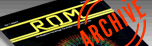ROM MAGAZINE ISSUE 5 — APRIL/MAY 1984 / PAGE 50`

 MISSILE MATH By TUONG (Tom) TRAN     Many times it is very hard to get a youngster to do any type of school work. The program below is a combination education-alarcade type game. The game requires the child to use both his brains and arcade skill to get a good high score. The game needs 16K and a joystick. In the game different math equations move across the top of your screen, and with your joystick you position your number shooter over the answer at the bottom of the screen and pull back. This will then pick up the number and display it on the bottom of your Number Shooter.     Once you have the correct answer displayed on your shooter, position the answer (with joy stick), directly under the equation. Then push the red button and joystick forward, and the number will be fired at the equation. If the answer is correct you'll hear a big explosion and ten points will be added to your score. If you are incorrect then part of the equation will break off and fall on your Number Shooter and destroy it. This will subtract one life and ten points from your score.     The game begins by giving you a few options. They are EQUAT (Equations, as many as you wish), LEVEL(Up to 15), LIVES(Up to 15), START(Begin game). Each option is selected or changed by the movement of the joystick. Once the flashing arrow is beside the option you want, press the red button. This will then allow you to change the option by pushing the joystick up(for higher) or down (for lower). The higher the level that you choose, the faster the equations cross the screen. This makes it very difficult, because the child has to first think of the correct answer and then pick the number up with the joystick.     This game was written entirely in BASIC without any assembler subroutines whatsoever. This program shows how easy it is to make a good educational tool without having to learn assembly language. If you have any changes that might make this program more enjoyable,I'd be happy to hear of them. Have fun and remember this game is for Kids!(Right?)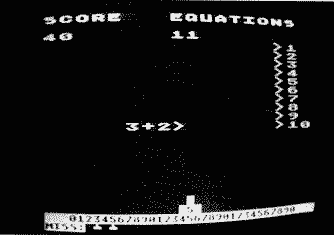10 REM ---BY--TOM--TUONG--TRAN--- 15 REM ---FOR ROM ISSUE 5-------- 20 DIM O\$(1),B(3),L(14):B(1)=20:B(2)=1:B(3)=1:F=128:FOR Q=0 TO 14:L(Q)=0:NEXT Q:GOTO 810 30 C=0:GRAPHICS 0:X=20:SCREEN=PEEK(88)+256*PEEK(89):P=SCREEN+X+40*21 40 GRAPHICS 1:POKE 710,96:SETCOLOR 2,0,0:POKE 752,1 50 ? "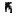↓":POKE 85,1:?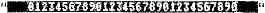:GOSUB 560 60 ? #6;"score   equations":? #6:? #6:LEVEL=1:FOR W=1 TO 10:? #6;"            >";W:NEXT W 70 GOSUB 80:GOTO 200 80 FOR Q=1 TO 50:NEXT Q:B(1)=B(1)-1:POSITION 9,2:? #6;"       ":POSITION 9,2:? #6;B(1):IF B(1)<=0 THEN 810 90 I=1:A=INT(RND(0)*6):B=INT(RND(0)*5):RN=INT(RND(0)*2):IF RN=0 THEN D=A+B:O\$="+":RETURN 100 IF B>A THEN TE=A:A=B:B=A 110 D=A-B:O\$="-":RETURN 120 FOR PO=SD TO SD+27 STEP 5:VOL=14-PO/20:SOUND 0,PO,0,VOL:SOUND 1,PO,8,VOL:SOUND 2,PO+15,2,VOL:NEXT PO 130 SD=SD+20:RETURN 150 POSITION 1,13-LEVEL:SOUND 1,70-I-B(2)*2,8,B(2)/15*2+0.5 160 POKE 85,I:? #6;"    ";:I=I+1 170 POKE 85,I:? #6;A;O\$;B;">"; 180 IF I>=13 THEN POKE 85,I-1:? #6;"    ";:SCORE=SCORE-(11-LEVEL):SOUND 1,0,0,0:GOSUB 80:GOTO 470 190 RETURN 200 GOSUB 150 210 FOR Q=1 TO 15-B(2):GOSUB 310:NEXT Q 220 GOTO 200 230 IF T=11 THEN X=X-1:GOTO 260 240 IF T=7 THEN X=X+1:GOTO 260 250 IF T=13 THEN F=PEEK(P+40) 260 IF X<=1 THEN X=X+1 270 IF X>=38 THEN X=X-1:RETURN 280 POKE P+1,0:POKE P-1,0:POKE P,0:POKE P-40,0:P=SCREEN+X+40*21 290 POKE P-1,128:POKE P,F:POKE P+1,128:POKE P-40,128 300 RETURN 310 IF STRIG(0)=0 AND C=0 THEN H=P-60+(20-INT(X/2+0.5)):GOSUB 150:GOSUB 340 320 T=STICK(0):IF T<>15 THEN GOSUB 230:RETURN 330 FOR Q=1 TO 250-B(2)*12:NEXT Q:RETURN 340 SD=20:SOUND 1,0,0,0 350 C=C+1:H=H-20:SOUND 0,SD,4,5:SD=SD+5 360 IF PEEK(H)<>0 THEN SD=5:GOTO 400 370 IF C>20 THEN GOSUB 480:C=0:SOUND 0,0,0,0:RETURN 380 POKE H,F-128 390 GOTO 340 400 REM 410 SOUND 0,0,0,0:GOSUB 480:IF D=F-128-16 THEN 430 420 GOSUB 490:GOTO 200 430 GOSUB 570 440 POKE 85,I-1:? #6;"    ";:GOSUB 80 450 SCORE=SCORE+10*LEVEL:IF SCORE>100*LEVEL AND LEVEL<10 THEN LEVEL=LEVEL+1 460 IF B(2)<15 AND SCORE>1000*B(2) AND LEVEL>=10 THEN B(2)=B(2)+1:LEVEL=1 470 C=0:POSITION 1,2:? #6;"    ":POSITION 1,2:? #6;SCORE:RETURN 480 FOR W=C TO 1 STEP -1:POKE H+W*20,0:NEXT W:RETURN 490 A1=PEEK(H):FOR W,=1 TO 200:NEXT W 500 FOR W=0 TO C-1:POKE H+20*W,A1 510 FOR Q=X1 TO X1+10:X1=X1+10:SOUND 0,Q,10,8:NEXT Q:X1=0 520 POKE H+20*W,0:NEXT W:GOSUB 800 530 POKE P,0:POKE P-40,0:POKE 85,I-1:? #6;"     ":C=0 540 FOR W=1 TO 10:POKE P+W,128:POKE P-W,128:GOSUB 120:POKE P+W,0:POKE P-W,0:NEXT W:GOSUB 600 550 ? "↑":?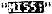:FOR Q=1 TO B(3)":? "  ";:NEXT Q:? "↑":B(3)=B(3)-1:IF B(3)<=0 THEN 810 560 ?;:FOR Q=1 TO B(3):? "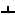";:NEXT Q:POKE P,F:POKE P-40,128:POKE P+1,128:POKE P-1,128:I=1:RETURN 570 FOR W=0 TO 5 580 GOSUB 800 590 NEXT W 600 FOR Q=0 TO 3:SOUND Q,0,0,0:NEXT Q:SD=0:RETURN 610 GRAPHICS 2+16:S=PEEK(88)+PEEK(89)*256+20*5+1:? #6;" ****************"; 620 ? #6;"** ":? #6;"    missile-math":? #6:? #6;" BY: TOM TUONG TRAN ":? #6:? #6;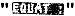;B(1) 630 POKE S+20,30:? #6;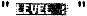;B(2):? #6;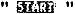:";B(3):? #6;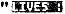";:? #6:? #6;"******************":C=1 640 IF STRIG(0)=0 THEN 690 650 T=STICK(0):IF T=15 THEN 640 660 POKE S+C*20,0:IF T=14 AND C>1 THEN C=C-1:GOTO 680 670 IF T=13 AND C<4 THEN C=C+1 680 POKE S+C*20,30:FOR Q=1 TO 50:NEXT Q:GOTO 640 690 IF C=4 THEN V1=B(1):V2=B(2):V3=B(3):GOTO 30 700 FOR Q=S+C*20+1 TO S+C*20+7:POKE Q,PEEK(Q)-128:NEXT Q:FOR Q=1 T0 100:NEXT Q:S2=0 710 IF STRIG(0)=0 THEN 790 720 T=STICK(0):IF T=15 THEN 710 730 S1=0:IF T=14 THEN S1=1 740 IF T=13 AND B(C)>0 THEN S1=-1 750 IF C=1 AND (B(1)>=16 OR T=14)THEN 780 760 IF C>1 AND (B(C)<=14 OR S1=-1) THEN 780 770 GOTO 710 780 B(C)=B(C)+S1:POSITION 9,5+C:?#6;"     ":POSITION 9,5+C:? #6;B(C):FOR Q=1 TO 20:NEXT Q:GOTO 720 790 FOR Q=S+C*20+1 TO S+C*20+7:POKE Q,PEEK(Q)+128:NEXT Q:FOR Q=1 T0 100:NEXT Q:GOTO 640 800 SETCOLOR 2,3,3:SETCOLOR 4,3,3:POKE 755,4:GOSUB 120:SETCOLOR 2,0,0:SETCOLOR 4,0,0:POKE 755,2:GOSUB 120:RETURN 810 GRAPHICS 1+16:IF B(1)<15 THEN B(1)=16 820 ? #6;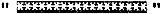:? #6;" missile-math":? #6:? #6:? #6;"2+5-4+3-2+1-8+ ":? #6:? #6 830 ? #6;:? #6:? #6;" BY:TOM TUONG TRAN":? #6 840 IF SCORE>HI THEN HI=SCORE:H1=HI:C1=1:GOSUB 920 850 ? #6:? #6;"      HI-SCORE":?#6: POKE 85,(20-C1)/2:? #6;HI:? #6:? #6;"  SCORE:";SCORE:? #6;" EQUATIONS:";B(1) 860 ? #6;"  LEVEL:";B(2):? #6;" LIVES:";B(3):? #6:? #6;" ******************" 870 IF STRIG(0)=0 THEN 610 880 GOSUB 940:POSITION 2,5:? #6;L(1);"+";(2);"-";L(3);"+"";L(4);"-";L(5);"+";L(6);"-";L(7);"+";L(8) 890 FOR Q=1 TO 50:NEXT Q 900 POSITION 2,5:? #6;"-";L(1);"+";L(2);"-";L(3);"+";L(4);"-";L(5);"+";L(6);"-";L(7);"+":FOR Q=1 TO 50:NEXT Q 910 GOTO 870 920 H1=H1/10:IF H1<1 THEN RETURN 930 C1=C1+1:GOTO 920 940 FOR Q=8 TO 1 STEP -1:L(Q)=L(Q-1):NEXT Q:L(1)=INT(RND(0)*10):RETURN